# PID Controller

•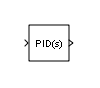## 描述

PID Controller 模块实现一个 PID 控制器（PID、PI、PD、仅 P 或仅 I）。该模块与时域参数设置为连续时间Discrete PID Controller 模块相同。

• 控制器类型（PID、PI、PD、仅 P 或仅 I）- 请参阅控制器参数。

• 控制器形式（Parallel 或 Ideal）- 请参阅形式参数。

• 时域（连续或离散）- 请参阅时域参数。

• 初始条件和重置触发器 - 请参阅外部重置参数。

• 输出饱和界限和内置抗饱和机制 - 请参阅限制输出参数。

• 无扰动切换和多环控制的信号跟踪 - 请参阅启用跟踪模式参数。

### 控制配置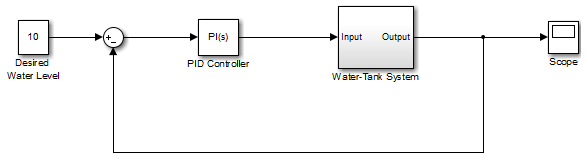### PID 增益调整

PID 控制器系数可手动或自动调整。自动调整需要 Simulink® Control Design™ 软件。有关自动调整的详细信息，请参阅选择调节方法参数。

## 端口

### 输入#### 依存关系

PID 调节工具，如 PID 调节器Closed-Loop PID Autotuner 模块，可调节增益 I，但无法调节增益 I*T s。因此，将从调节工具获得的积分增益值乘以采样时间，然后将其提供给此端口。

#### 依存关系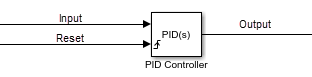#### 依存关系

`$D\left[\frac{N}{1+N\alpha \left(z\right)}\right],$`

`$\alpha \left(z\right)=\frac{{T}_{s}}{z-1}.$`

`$\alpha \left(z\right)=\frac{{T}_{s}z}{z-1}.$`

`$\alpha \left(z\right)=\frac{{T}_{s}}{2}\frac{z+1}{z-1}.$`

## 参数

PID

PI

PD

P

I

#### 编程用法

 模块参数：`Controller` 类型：字符串、字符向量 值：`"PID"`、`"PI"`、`"PD"`、`"P"`、`"I"` 默认值：`"PID"`

`${C}_{par}\left(s\right)=P+I\left(\frac{1}{s}\right)+D\left(\frac{Ns}{s+N}\right).$`

`${C}_{par}\left(z\right)=P+I\alpha \left(z\right)+D\left[\frac{N}{1+N\beta \left(z\right)}\right],$`

`${C}_{id}\left(s\right)=P\left[1+I\left(\frac{1}{s}\right)+D\left(\frac{Ns}{s+N}\right)\right].$`

`${C}_{id}\left(z\right)=P\left[1+I\alpha \left(z\right)+D\frac{N}{1+N\beta \left(z\right)}\right],$`

#### 编程用法

 模块参数：`Controller` 类型：字符串、字符向量 值：`"Parallel"`、`"Ideal"` 默认值：`"Parallel"`

PID Controller 模块位于具有同步状态控件（请参阅 State Control (HDL Coder) 模块）的模型中时，您不能选择连续时间

PID ControllerDiscrete PID Controller 模块是相同的，只是此参数的默认值不同。

#### 编程用法

 模块参数：`TimeDomain` 类型：字符串、字符向量 值：`"Continuous-time"`、`"Discrete-time"` 默认值：`"Continuous-time"`

#### 编程用法

 模块参数：`UseExternalTs` 类型：字符串、字符向量 值：`"on"`、`"off"` 默认值：`"off"`

#### 编程用法

 模块参数：`SampleTime` 类型：标量 值： `-1`、正标量 默认值： `-1`

`$\alpha \left(z\right)=\frac{{T}_{s}}{z-1}.$`

`$\alpha \left(z\right)=\frac{{T}_{s}z}{z-1}.$`

`$\alpha \left(z\right)=\frac{{T}_{s}}{2}\frac{z+1}{z-1}.$`

• 选择了限制输出抗饱和方法以外的值。

• 选择了启用跟踪模式

#### 编程用法

 模块参数：`IntegratorMethod` 类型：字符串、字符向量 值：`"Forward Euler"`、`"Backward Euler"`、`"Trapezoidal"` 默认值：`"Forward Euler"`

`$D\left[\frac{N}{1+N\alpha \left(z\right)}\right],$`

`$\alpha \left(z\right)=\frac{{T}_{s}}{z-1}.$`

`$\alpha \left(z\right)=\frac{{T}_{s}z}{z-1}.$`

`$\alpha \left(z\right)=\frac{{T}_{s}}{2}\frac{z+1}{z-1}.$`

#### 编程用法

 模块参数：`FilterMethod` 类型：字符串、字符向量 值：`"Forward Euler"`、`"Backward Euler"`、`"Trapezoidal"` 默认值：`"Forward Euler"`

### 常设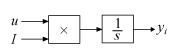`${y}_{i}=\int uI\text{\hspace{0.17em}}dt.$`

#### 编程用法

 模块参数：`ControllerParametersSource` 类型：字符串、字符向量 值：`"internal"`、`"external"` 默认值：`"internal"`

• 并行 - 比例动作独立于积分和导数动作。例如，对于连续时间并行 PID 控制器，传递函数为：

`${C}_{par}\left(s\right)=P+I\left(\frac{1}{s}\right)+D\left(\frac{Ns}{s+N}\right).$`

对于离散时间并行形式的控制器，传递函数为：

`${C}_{par}\left(z\right)=P+I\alpha \left(z\right)+D\left[\frac{N}{1+N\beta \left(z\right)}\right],$`

其中积分器方法滤波器方法参数分别确定 α(z) 和 β(z)。

• 理想 - 比例增益乘以积分和导数项。例如，对于连续时间理想 PID 控制器，传递函数为：

`${C}_{id}\left(s\right)=P\left[1+I\left(\frac{1}{s}\right)+D\left(\frac{Ns}{s+N}\right)\right].$`

对于离散时间理想形式的控制器，传递函数为：

`${C}_{id}\left(z\right)=P\left[1+I\alpha \left(z\right)+D\frac{N}{1+N\beta \left(z\right)}\right],$`

其中积分器方法滤波器方法参数分别确定 α(z) 和 β(z)。

#### 编程用法

 模块参数：`P` 类型：标量、向量 默认值：1

#### 编程用法

 模块参数：`I` 类型：标量、向量 默认值：1

PID 调节工具，如 PID 调节器Closed-Loop PID Autotuner 模块，可调节增益 I，但无法调节增益 I*T s。因此，将从调节工具获得的积分增益值乘以采样时间，然后将其写入此参数。

#### 编程用法

 模块参数：`I` 类型：标量、向量 默认值：1

#### 编程用法

 模块参数：`UseKiTs` 类型：字符串、字符向量 值：`"on"`、`"off"` 默认值：`"on"`

#### 编程用法

 模块参数：`D` 类型：标量、向量 默认值：0

`$D\frac{z-1}{z{T}_{s}}.$`

#### 编程用法

 模块参数：`UseFilter` 类型：字符串、字符向量 值：`"on"`、`"off"` 默认值：`"on"`

• 时域连续时间时，极点位置为 `s = -N`

• 时域离散时间时，极点位置取决于滤波器方法参数。

滤波器方法滤波器极点的位置
前向欧拉${z}_{pole}=1-N{T}_{s}$
后向欧拉${z}_{pole}=\frac{1}{1+N{T}_{s}}$
梯形${z}_{pole}=\frac{1-N{T}_{s}/2}{1+N{T}_{s}/2}$

#### 编程用法

 模块参数：`N` 类型：标量、向量 默认值：100

• 在初始状态重置时

• 在进入上限或下限饱和状态时

• 在退出上限或下限饱和状态时

#### 编程用法

 模块参数：`ZeroCross` 类型：字符串、字符向量 值：`"on"`、`"off"` 默认值：`"on"`

### 初始化：

#### 编程用法

 模块参数：`InitialConditionSource` 类型：字符串、字符向量 值：`"internal"`、`"external"` 默认值：`"internal"`

#### 编程用法

 模块参数：`InitialConditionForIntegrator` 类型：标量、向量 默认值：0

#### 编程用法

 模块参数：`InitialConditionForFilter` 类型：标量、向量 默认值：0

#### 编程用法

 模块参数：`DifferentiatorICPrevScaledInput` 类型：标量、向量 默认值：0

#### 编程用法

 模块参数：`InitialConditionSetting` 类型：字符串、字符向量 值：`"Auto"`、`"Output"` 默认值：`"Auto"`

• 在当前时间步为非零值时

• 从上一时间步的非零更改为当前时间步的零时

#### 编程用法

 模块参数：`ExternalReset` 类型：字符串、字符向量 值：`"none"`、`"rising"`、`"falling"`、`"either"`、`"level"` 默认值：`"none"`

#### 编程用法

 模块参数：`IgnoreLimit` 类型：字符串、字符向量 值：`"off"`、`"on"` 默认值：`"off"`

#### 无扰动切换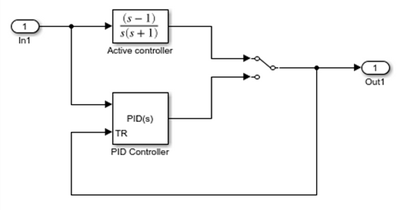#### 多回路控制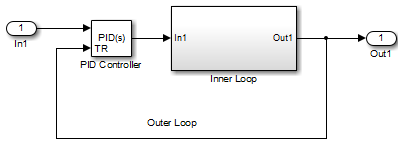Inner Loop 子系统包含下图所示的模块。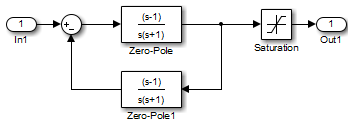#### 编程用法

 模块参数：`TrackingMode` 类型：字符串、字符向量 值：`"off"`、`"on"` 默认值：`"off"`

#### 编程用法

 模块参数：`Kt` 类型：标量 默认值：1

### 饱和

#### 编程用法

 模块参数：`LimitOutput` 类型：字符串、字符向量 值：`"off"`、`"on"` 默认值：`"off"`

#### 编程用法

 模块参数：`SatLimitsSource` 类型：字符串、字符向量 值：`"internal"`、`"external"` 默认值：`"internal"`

#### 编程用法

 模块参数：`UpperSaturationLimit` 类型：标量 默认值：`Inf`

#### 编程用法

 模块参数：`LowerSaturationLimit` 类型：标量 默认值：`-Inf`

#### 编程用法

 模块参数：`LinearizeAsGain` 类型：字符串、字符向量 值：`"off"`、`"on"` 默认值：`"off"`

• 输入信号的符号永远不变，积分器继续计算积分，直到溢出。溢出值是积分器输出的数据类型的最大值或最小值。

• 如果输入信号的符号在加权和超出输出界限时发生改变，它将需要很长时间来防止积分器饱和，并返回位于模块饱和界限范围内的加权和。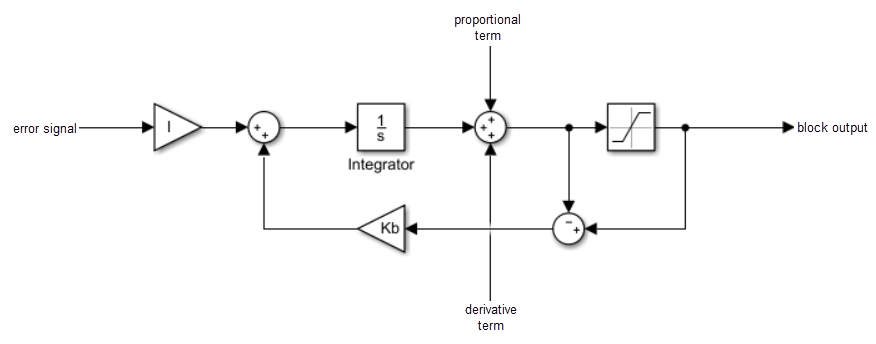#### 编程用法

 模块参数：`AntiWindupMode` 类型：字符串、字符向量 值：`"none"`、`"back-calculation"`、`"clamping"` 默认值：`"none"`

#### 编程用法

 模块参数：`Kb` 类型：标量 默认值：1

#### 编程用法

 模块参数：`LimitIntegratorOutput` 类型：字符串、字符向量 值：`"off"`、`"on"` 默认值：`"off"`

#### 编程用法

 模块参数：`UpperIntegratorSaturationLimit` 类型：标量 默认值：`Inf`

#### 编程用法

 模块参数：`LowerIntegratorSaturationLimit` 类型：标量 默认值：`-Inf`

### 数据类型

• 浮点或定点数据类型，包括数据类型是否继承自模块中的上游值。

• 量的最小值和最大值，用于确定如何对量进行定标以使用定点表示。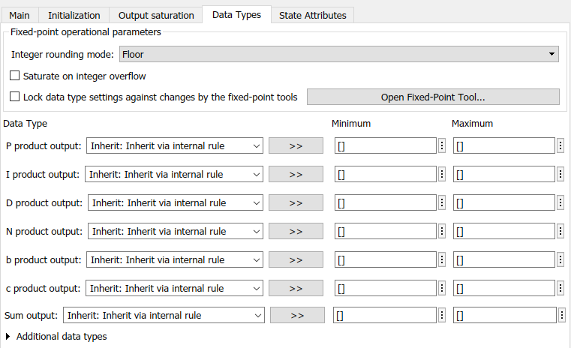“数据类型”选项卡中具体列出的量取决于您配置 PID 控制器模块的方式。通常，您可以为以下类型的量配置数据类型：

• 乘积输出 - 存储在模块封装下执行的乘法的结果。例如，P 乘积输出存储增益模块的输出，该输出是模块输入与比例增益 P 的乘积。

• 参数 - 存储数值模块参数的值，例如 PID

• 模块输出 - 存储位于 PID 控制器模块封装下的模块的输出。例如，使用积分器输出指定名为 Integrator 的模块输出的数据类型。此模块位于 Integrator 子系统中的封装下，并计算控制器操作的积分项。

• 累加器 - 存储与求和模块关联的值。例如，SumI2 累加器设置与求和模块 SumI2 关联的累加器的数据类型。此模块位于 Anti-Windup 子系统的 Back Calculation 子系统中的封装下。

#### 编程用法

 模块参数：`RndMeth` 类型：字符向量 值：`'Ceiling' | 'Convergent' | 'Floor' | 'Nearest' | 'Round' | 'Simplest' | 'Zero'` 默认值：`'Floor'`

• `off` - 溢出将绕回到数据类型可以表示的合适值。

例如，数字 130 不适合一个有符号的 8 位整数，因此绕回 -126。

• `on` - 将溢出饱和处理为数据类型能够表示的最小值或最大值。

例如，一个有符号的 8 位整数的溢出可以饱和处理为 -128 或 127。

• 如果您的模型存在可能的溢出，而您希望在生成的代码中进行显式饱和保护，请考虑选中此复选框。

• 如果您希望优化生成的代码的效率，请考虑清除此复选框。

清除此复选框还可以帮助您避免过度地指定信号超出范围时的处理方式。有关详细信息，请参阅信号范围错误故障排除

• 如果选中此复选框，饱和将应用于模块中的每个内部操作，而不仅仅应用于输出或结果。

• 一般情况下，代码生成进程可以检测到何时不可能发生溢出。在这种情况下，代码生成器不会生成饱和代码。

#### 编程用法

 模块参数：`SaturateOnIntegerOverflow` 类型：字符向量 值：`'off' | 'on'` 默认值：`'off'`

#### 编程用法

 模块参数：`LockScale` 类型：字符向量 值：`'off' | 'on'` 默认值：`'off'`

### 状态属性

• 用于生成代码中的对应变量

• 作为在仿真期间记录状态时存储名称的一部分

• 用于通过线性化模块获得的线性模型中的对应状态

#### 编程用法

 参数：`IntegratorContinuousStateAttributes`、`FilterContinuousStateAttributes` 类型：字符向量 默认值：`''`

• 用于生成代码中的对应变量

• 作为在仿真期间记录状态时存储名称的一部分

• 用于通过线性化模块获得的线性模型中的对应状态

#### 编程用法

 参数：`IntegratorStateIdentifier`、`FilterStateIdentifier` 类型：字符串、字符向量 默认值：`""`

#### 依存关系

1. 时域设置为离散时间

2. 指定积分器或滤波器状态名称的值。

3. 将模型配置参数信号解析设置为以外的值。

#### 编程用法

 模块参数：`IntegratorStateMustResolveToSignalObject`、`FilterStateMustResolveToSignalObject` 类型：字符串、字符向量 值：`"off"`、`"on"` 默认值：`"off"`

## 模块特性

 数据类型 `double` | `fixed point` | `integer` | `single` 直接馈通 `否` 多维信号 `否` 可变大小信号 `否` 过零检测 `否`

 Visioli, A., "Modified Anti-Windup Scheme for PID Controllers," IEE Proceedings - Control Theory and Applications, Vol. 150, Number 1, January 2003